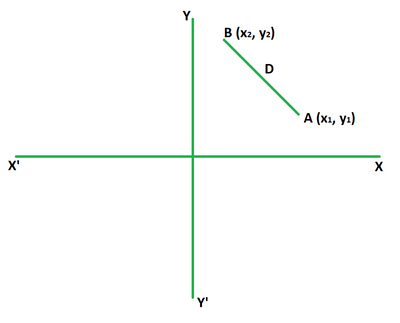GeeksforGeeks App
Open AppBrowser
Continue

# How to find the equidistant points on the Y axis?

The distance formula is used to calculate the distance between any two points in a two-dimensional or three-dimensional plane. In other words, it gives the distance between two different locations on a cartesian plane.

### What is the Distance Formula?

It applies the Pythagorean theorem to determine the required distance. Its formula claims that the distance between any two coordinates is equal to the square root of the differences between the x-coordinates and y-coordinates of the points. It is used to evaluate the distance between point to point, point to plane, and plane to plane.D = √((x2 – x1)2 + (y2 – y1)2)

where,

• D is the distance between the points,
• (x1, y1) and (x2, y2) are the coordinates.

### How to find the equidistant points on the Y-axis?

Consider two points A (a, b) and B (p, q) lying at a distance from each other on a two dimensional plane.

We have to find a point on the y-axis which is equidistant from these points. It is known that any point that lies on y-axis is of the form (0, y).

Suppose C is (0, y). According to the problem we can conclude that,

AC = BC

AC2 = BC2

Using distance formula we have,

(0 – a)2 + (y – b)2 = (0 – p)2 + (y – q)2

a2 + y2 + b2 – 2yb = p2 + y2 + q2 – 2yq

2y (q – b) = p2 – q2 – a2 – b2

y = (p2 – q2 – a2 – b2)/2(q – b)

The above value is calculated by substituting the given values of a, b, p and q. This gives us the point required (0, y).

### Sample Problems

Problem 1: Find the point on the y-axis which is equidistant from (-3, 4) and (5, 2).

Solution:

Suppose the required point is (0, y).

Using distance formula we get,

(-3 – 0)2 + (4 – y)2 = (5 – 0)2 + (2 – y)2

9 + 16 + y2 – 8y = 25 + 4 + y2 – 4y

-8y + 4y – 4 = 0

4y = -4

y = -1

So, the required point is (0, -1).

Problem 2: Find the point on the y-axis which is equidistant from (6, 3) and (4, 1).

Solution:

Suppose the required point is (0, y).

Using distance formula we get,

(6 – 0)2 + (3 – y)2 = (4 – 0)2 + (1 – y)2

36 + 9 + y2 – 6y = 16 + 1 + y2 – 2y

45 – 6y – 17 + 2y = 0

4y = 28

y = 7

So, the required point is (0, 7).

Problem 3: Find the point on the y-axis which is equidistant from (3, 2) and (8, 4).

Solution:

Suppose the required point is (0, y).

Using distance formula we get,

(3 – 0)2 + (2 – y)2 = (8 – 0)2 + (4 – y)2

9 + 4 + y2 – 4y = 64 + 16 + y2 – 8y

13 – 4y – 80 + 8y = 0

4y = 67

y = 67/4

So, the required point is (0, 67/4).

Problem 4: Find the point on the y-axis which is equidistant from (5, 1) and (7, 2).

Solution:

Suppose the required point is (0, y).

Using distance formula we get,

(5 – 0)2 + (1 – y)2 = (7 – 0)2 + (2 – y)2

25 + 1 + y2 – 2y = 49 + 4 + y2 – 4y

26 – 2y – 53 + 4y = 0

2y = 27

y = 27/2

So, the required point is (0, 27/2).

Problem 5: Find the value of x if (0, 3) is equidistant from (x, 5) and (3, 6).

Solution:

Using the distance formula we get,

(x – 0)2 + (5 – 3)2 = (3 – 0)2 + (6 – 3)2

x2 + 4 = 9 + 9

x2 = 18 – 4

x2 = 14

x = ±3.74

Problem 6: Find the value of x if (0, 2) is equidistant from (x, 1) and (5, 2).

Solution:

Using the distance formula we get,

(x – 0)2 + (1 – 2)2 = (5 – 0)2 + (2 – 2)2

x2 + 1 = 25 + 0

x2 = 25 – 1

x2 = 24

x = ±4.89

Problem 7: Find the value of x if (0, 6) is equidistant from (x, 3) and (7, 4).

Solution:

Using the distance formula we get,

(x – 0)2 + (3 – 6)2 = (7 – 0)2 + (4 – 6)2

x2 + 9 = 49 + 4

x2 = 53 – 9

x2 = 44

x = ±6.63

My Personal Notes arrow_drop_up
Related Tutorials